# Print ggplot2 Plot within for-Loop in R (Example)

In this article you’ll learn how to draw ggplot2 plots within a for-loop in the R programming language.

The post looks as follows:

You’re here for the answer, so let’s get straight to the programming part…

## Introducing Example Data

We will use the following data as basement for the example of this R programming tutorial:

```set.seed(159159)                                      # Create example data
data <- data.frame(x = 1:100,
y1 = rnorm(100),
y2 = rnorm(100),
y3 = rnorm(100))```

Our example data contains 100 rows and four columns. The variable x is ranging from 1 to 100 and the columns y1, y2, and y2 contain 100 random normally distributed numbers each.

We also need to install and load the ggplot2 add-on package to RStudio:

```install.packages("ggplot2")                           # Install and load ggplot2
library("ggplot2")```

Now, we can draw a basic ggplot2 graph as follows:

```ggplot(data, aes(x = x, y = y1)) +                    # Basic ggplot2 plot of x & y1
geom_point()```Figure 1: Basic Scatterplot Created by ggplot2 Package.

In Figure 1, you can see the result of the previous R code: A scatterplot of x and y1.

Now, let’s assume we want to create a ggplot2 plot of each combination of x and y1, y2, and y3 respectively. In such a scenario, we may want to use a for-loop:

```for(i in 2:ncol(data)) {                              # ggplot within for-loop
ggplot(data, aes(x = x, y = data[ , i])) +
geom_point()
Sys.sleep(2)
}```

Damn! No graphic was returned!

So why does ggplot2 not work if it is inside a for-loop although it works outside of it?!

That’s what I’m going to show you next…

## Example: Plotting ggplot2 Plots within Loop

If we want to draw a plot within a for-loop, we need to wrap the print function around the R code creating the plot. Have a look at the following R syntax:

```for(i in 2:ncol(data)) {                              # Printing ggplot within for-loop
print(ggplot(data, aes(x = x, y = data[ , i])) +
geom_point())
Sys.sleep(2)
}```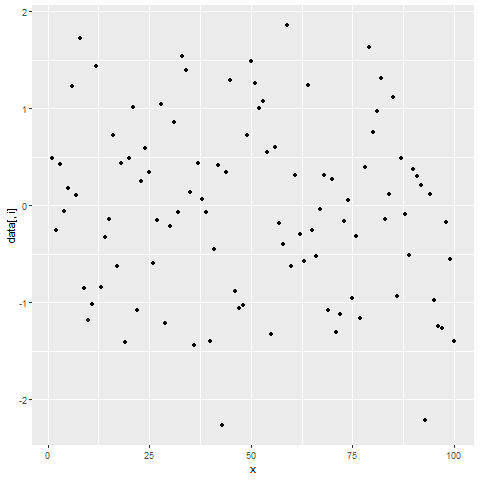Figure 2: Showing ggplot2 Plots within for-Loop using print() Function.

After running the previous R code, you will see three ggplot2 graphs popping up at the bottom right of RStudio with a delay of 2 seconds.

Looks good!

## Video, Further Resources & Summary

Would you like to know more about the ggplot2 package in R? Then you could watch the following video of my YouTube channel. In the video, I explain the R codes of this article in RStudio:

Please accept YouTube cookies to play this video. By accepting you will be accessing content from YouTube, a service provided by an external third party.If you accept this notice, your choice will be saved and the page will refresh.

Furthermore, you might have a look at the related articles of this website. A selection of articles can be found here.

Subscribe to the Statistics Globe Newsletter

•Anoop
January 8, 2021 10:57 am

Hi , Thanks for the tutorial on ggplot loop! Is there a way to dynamically assign the variable names as Y-Axis labels?

•January 8, 2021 12:18 pm

Hi Anoop,

Thank you for the comment.

Please have a look at the following tutorials:

They explain how to assign names dynamically. You can also use this logic to assign Y-axis labels dynamically.

Regards,

Joachim

•BB
June 8, 2022 11:12 am

Hi Joachim
This was an excellent description of how to create the for loop for ggplotting.

I have a follow up question related to Anoop’s question that I hope you can help with, because I don’t seem to be able to work it out from the links you have shared.

I would like to assign my column names to be the x labels (my y variable is always the same), but when I try with the code that I put together from the links you shared I only ever get the column number and not the column name. I have tried passing a character list of column names, however it only ever uses the first name in the list.

Can you help clear this up a little for me please, as I am not sure where I am going wrong.

•June 8, 2022 11:18 am

Hey,

Thanks a lot for the kind comment, it’s great to hear that you find this tutorial helpful!

Regards,
Joachim

•BB
June 8, 2022 1:46 pm

Thank you.
I have a dataframe of 36 observations of 17 variables, but in this iteration of what I am doing I only need to plot column 2 (Total_Erosion) against columns 8-17.

Am using the code below at the moment:
for (i in 8:ncol(Bank1Variables)) {
print(ggplot(data = Bank1Variables, aes_string(x = Bank1Variables[ , i], y = Total_Erosion*-1)) +
geom_point() +
labs(x = assign(paste0(” “, i), value = i), y = names(Bank1Variables)) +
geom_smooth(method = “lm”, se = FALSE))
}

Which produces my plots beautifully, and names my Y axis correctly but my X axes are only ever named based on their row number and not their name.

I have also created a character vector of the column names that I am using

w <- c("Mean_Stage", "Max_Stage", "Peaks_AboveQ10", "Peaks_AboveQ50", "Hours_AboveQ10", "Hours_AboveQ50")

But when I include the vector in the code as follows:
for (i in 8:ncol(Bank1Variables)) {
print(ggplot(data = Bank1Variables, aes_string(x = Bank1Variables[ , i], y = Total_Erosion*-1)) +
geom_point() +
labs(x = assign(paste0(" ", w), value = w), y = names(Bank1Variables)) +
geom_smooth(method = "lm", se = FALSE))
}

It only ever uses the first name of Mean_Stage and labels all plots the same and then if i replace the w with w[i] it labels them all as NA

It's likely to be something extremely simply and silly, but I have been working on it for a while now and can't get it to work.

Thank you for your time. I really appreciate it!

•June 8, 2022 3:44 pm

Thanks for the clarifications! You may simply add the xlab and ylab functions to your ggplot2 code.

Have a look at the following example. It shows how to modify the yaxis label dynamically:

```for(i in 2:ncol(data)) {
print(ggplot(data, aes(x = x, y = data[ , i])) +
geom_point()) +
ylab(colnames(data)[i]) # Change ylab
Sys.sleep(2)
}```

Let me know in case you need further help! 🙂

Regards,
Joachim

•Moh
June 9, 2021 5:54 am

Hi,
I am getting following error.

Error in data[, i] : object of type ‘closure’ is not subsettable.

•Ale
June 17, 2021 8:16 am

Hi and thanks for the tutorial! Is there a way to show all graphs at the same time? In R, without using a pdf (it’s only 9 graphs for me)

•Christer P. Volk
August 12, 2021 8:37 am

Hi Joachim

This is great. Been having this issue a few times. But why does it work? What does print() do that is needed here?

•August 12, 2021 10:21 am

Hi Christer,

Thank you for the nice comment, glad it helped!

You can read more about the reasons in this article, specifically: “An interesting side-effect of this is that ggplots are only rendered when explicitly print()ed/plot()ed within a loop, as only the last return value in a sequence of calls gets its print method invoked.”

Regards

Joachim

•Richard Ngaya
September 17, 2021 6:10 am

THis website was very helpful for me. I have always tried several times to learn creating loops in R and the books have not been good. But, your website made me like to go through all your examples and even try some other new options just to see how the outputs changes.

Thank you very much!!!!!!!!!!!!!!!!!!!!!!!!!

•September 17, 2021 6:12 am

Thanks a lot for this wonderful feedback Richard! I’m very glad to hear that my tutorials helped to improve your R programming skills 🙂

Regards

Joachim

•Littleag
September 19, 2021 5:56 pm

I spent days on this problem, but with your help it will be a big time-saver in the future! I especially like how you showed the initial lack of result (“Damn! No graphic…”), just as I experienced it (many times). I can’t thank you enough.

•September 20, 2021 6:18 am

Thanks a lot for the kind comment Littleag! Glad it helped! 🙂

Regards

Joachim

•JOE
September 30, 2021 8:54 am

HI ！
i fellowed your code ,and it work fine, when i try to save each plot of them,i meet so error.
———————————————————————————————————————————
for (i in 2:ncol(data)) {
p=( ggplot(data=data) + geom_bar(aes(x=tissue,y=data[,i]),stat=”identity”)+theme_classic())
plot_list[[i]] = p}
———————————————————————————————————————————
Rstudio did not repot error,but the plot was not the plot when i use code as you descripted
————————————————————————————————————————
for (i in 2:ncol(data)) {

print( ggplot(data=data) + geom_bar(aes(x=tissue,y=data[,i]),stat=”identity”)+theme_classic())
Sys.sleep(2)}
——————————–
thanks u

•September 30, 2021 11:37 am

Hey Joe,

You mean you want to export these plots as PDF or PNG? I’m not certain if I get your question correctly.

Regards

Joachim

•Mike
January 15, 2022 4:54 am

I think that’s what the poster was asking, and it’s what I really want to know as well.

•January 17, 2022 7:40 am

Hey Mike,

You can export all plots as PDFs as shown below:

```for(i in 2:ncol(data)) {                              # Printing ggplot within for-loop
pdf(paste0("plot_no_", i - 1, ".pdf"))
print(ggplot(data, aes(x = x, y = data[ , i])) +
geom_point())
dev.off()
}```

I hope that helps!

Joachim

•Sameer Joshi
January 25, 2022 5:01 am

Hi Joachim, very useful script. How ever I have a question could you please answer this.

for(i in 2:ncol(data))

Can you please explain why you have put 2. Thanks

•January 25, 2022 6:48 am

Hey Sameer,

Thank you, glad it is useful!

The 2:ncol is used because the first column in our data frame is the x-variable, and we do only want to loop over the y-variables (i.e. positions 2:ncol(data)).

Regards,
Joachim

•Joshua
May 17, 2022 11:59 am

Hi Joachim,

I am using for-loops to produce multiple graphs in RMarkdown. I assign them to a list so that they are able to print or to be exported to html or word document. My problem is that i would like to use for-loops then export results of each single code chunk to a separate document i.e. 5 RMarkdown code chunks = 5 separate word documents. I can do this with officer package when the plots are not produced by for-loops or assigned to a list but if i do this for plots on a list produced by for-loop, i only get 1 plot only.

Is there a way to do this in a simple way?

•May 18, 2022 5:35 am

Hey Joshua,

Unfortunately, I’m not an expert on RMarkdown. However, could you share your code? I could have a look and try to fx it.

Regards,
Joachim

•BB
June 8, 2022 7:35 pm

Hi Joachim
For some reason I couldn’t reply to your last message on our thread but I just wanted to say that your solution worked perfectly.

I am so grateful for your help! Thank you for your time and effort.
It is much appreciated!
BB

•June 9, 2022 6:17 am

This is really great to hear, glad it was useful! 🙂

Regards,
Joachim

•Sam
August 8, 2022 2:53 pm

Thanks for the information – really helpful to learn so far!

I’d really like to be able to do the same steps, but have each plot combine into the same plot – is there a way to adapt this to work?

Specifically, I am trying to plot several normal distributions from a data.table onto the same plot. Any advice appreciated!

•August 9, 2022 6:36 am

Hey Sam,

Thanks for the kind comment, glad it’s useful.

Regarding your question, did you already have a look at the patchwork package? It’s a great package for combining multiple plots.

Regards,
Joachim

•Alice
August 26, 2022 8:29 am

Hi,
Thank you very much for your video. I tried to apply your code to my data with the following script :

for (i in 2:ncol(df)) {
print(ggplot(df, aes(x = Date_Time, y = df[ , i]))+
geom_point())
Sys.sleep(2)
}

But it’s sending an error message :
Error in `[.data.table`(df, , i) :
j (the 2nd argument inside […]) is a single symbol but column name ‘i’ is not found. Perhaps you intended DT[, ..i]. This difference to data.frame is deliberate and explained in FAQ 1.1.

I don’t understand why it’s not working, if it’s working on your session.

•August 29, 2022 9:25 am

Hey Alice,

It seems like you are working with a data.table instead of a data.frame. Could you please try if it works when you convert your data.table to the data.frame class first? See here for more details.

Regards,
Joachim

•Niels
September 16, 2022 10:10 am

Hi Joachim,

Thanks a lot for there instructions. I’m still running into a small problem with my own data.
I would like to create multiple plots, one for each of my participants.
So my dataframe looks like this:

x= subjectID
y=time point
z=concentration of a biomarker.

I want to create a plot of y vs. z, for every x. So i need to loop over x, but I cant figure out how to incorporate a third column in this code..

•September 19, 2022 11:38 am

Hi Niels,

Thank you for the kind comment.

Please have a look at the following example code. It reproduces your question:

```set.seed(8374636)
data <- data.frame(ID = 1:5,
time = rnorm(100),
marker = rnorm(100))

library("ggplot2")

for(i in 1:length(unique(data\$ID))) {
print(ggplot(data[data\$ID == unique(data\$ID)[i], ], aes(x = time, y = marker)) +
geom_point() +
ggtitle(paste("ID No.", i)))
Sys.sleep(2)
}```

Regards,
Joachim

•Niels
September 22, 2022 8:52 am

Hi Joachim,

thanks so much for your answer. I was able to adjust a little to fit my data and everything worked fine!
However, after this I tried to incorporate an earlier answer from you in order to save my plots as PDF. But this resulted in the following error message:

Error in grid.Call.graphics(C_text, as.graphicsAnnot(x\$label), x\$x, x\$y, :
invalid font type

I’ve tried several thing to fix this, all not working sadly. Strange thing now is when I remove all the PDF lines, my original code is also returning the same error…
Do you have any suggestions?

My complete code looks like this:

for(i in 1:length(unique(TropT\$Record_ID))) {
print(ggplot(TropT[TropT\$Record_ID == unique(TropT\$Record_ID)[i], ], aes(x = phase, y = Lab_TropT, group=1)) +
ggtitle(paste(TropT\$Record_ID == unique(TropT\$Record_ID)[i])) +
theme(plot.title = element_text(
face = “bold”,
colour = “#333333”,
size = 40,
hjust = 0))+
ylab(“Troponine T (ng/L)”) + theme(plot.title = element_text(
face = “bold”,
colour = “#333333”,
size = 20))+
xlab(“Meetmoment”) + theme(plot.title = element_text(
face = “bold”,
colour = “#333333”,
size = 20))+
geom_line(colour = “#333333″)+
geom_point(shape=21, color=”black”, fill=”#69b3a2″, size=6)+
scale_y_continuous(
limits = c(0, 50),
breaks = seq(from = 0, to = 50, by = 5))+
theme_ipsum())
Sys.sleep(2)
}

•Niels
September 22, 2022 9:13 am

Previous question includes the wrong code..
This is the correct version:

for(i in 1:length(unique(TropT\$Record_ID))) {
pdf(paste0(“TropT plots.pdf”))
print(ggplot(TropT[TropT\$Record_ID == unique(TropT\$Record_ID)[i], ], aes(x = phase, y = Lab_TropT, group=1)) +
ggtitle(paste(TropT\$Record_ID[i])) +
theme(plot.title = element_text(
face = “bold”,
colour = “#333333”,
size = 40,
hjust = 0))+
ylab(“Troponine T (ng/L)”) + theme(plot.title = element_text(
face = “bold”,
colour = “#333333”,
size = 20))+
xlab(“Meetmoment”) + theme(plot.title = element_text(
face = “bold”,
colour = “#333333”,
size = 20))+
geom_line(colour = “#333333″)+
geom_point(shape=21, color=”black”, fill=”#69b3a2″, size=6)+
theme_ipsum())
dev.off()
}

•September 30, 2022 4:56 pm

Hi Nils,

I’m sorry for the late reply, unfortunately I couldn’t respond earlier. Do you still need help with this error message?

Regards,
Joachim

•Niels
October 24, 2022 2:32 pm

Hi Joachim,

I would like to follow up on my previous questions with an new problem.
For data exploring, I would like to create multiple graphs, one for each Markers, showing the concentration of that marker on the Y axis, with time on the X-axis with multiple ID’s in the same graph.
somewhat example would be: https://i2.wp.com/michaeltoth.me/figures/20190416_ggplot_geom_line/color_aes-1.png?zoom=1.5&w=578&ssl=1

I can’t figure out how to create te loop. Dataset example would be:

set.seed(8374636)
data <- data.frame(ID = 1:5,
time = 1:5,
markerA = rnorm(100)),
markerB = rnorm(100),
markerC = rnorm(100),

ggplot(data, aes(x=time,
y=marker (A, B, C etc),
col=ID,
group=ID,
text = ID))+
geom_line(
size=1)+
geom_point(
size=2)

I won't bother you with the design part of the code, so I left that out.
Hope you can help me once again. Thanks in advance!

•November 14, 2022 12:41 pm

Hi Niels,

I’m sorry for the delayed response. I was on a long vacation, so unfortunately I wasn’t able to get back to you earlier. Do you still need help with your syntax?

Regards,
Joachim

•Niels van Steijn
November 14, 2022 1:34 pm

Hi Joachim,

•November 15, 2022 9:14 am

Hi Niels,

I’m uncertain if I got it correctly which component of your data should be shown at which position. However, I have created an updated example, which hopefully helps to find a solution for your situation.

I think the crucial point of the following code is that I’m reshaping the data from wide to long format using the pivot_longer function.

Consider the example code and its resulting graph below:

```set.seed(8374636)
data <- data.frame(time = 1:100,
markerA = rnorm(100, 0),
markerB = rnorm(100, 3),
markerC = rnorm(100, 6))
#   time    markerA  markerB  markerC
# 1    1  1.9593895 2.635747 6.346182
# 2    2  1.3040143 2.681916 6.354147
# 3    3  0.2675190 4.877706 4.980549
# 4    4 -0.8246069 2.466270 6.916204
# 5    5 -0.6764416 2.374901 6.347952
# 6    6 -1.3834653 2.074864 5.668141

library("tidyr")

data_long <- pivot_longer(data, cols = c("markerA", "markerB", "markerC"))
# # A tibble: 6 x 3
#    time name    value
#   <int> <chr>   <dbl>
# 1     1 markerA  1.96
# 2     1 markerB  2.64
# 3     1 markerC  6.35
# 4     2 markerA  1.30
# 5     2 markerB  2.68
# 6     2 markerC  6.35

library("ggplot2")

ggplot(data_long, aes(x = time, y = value, col = name)) +
geom_line()```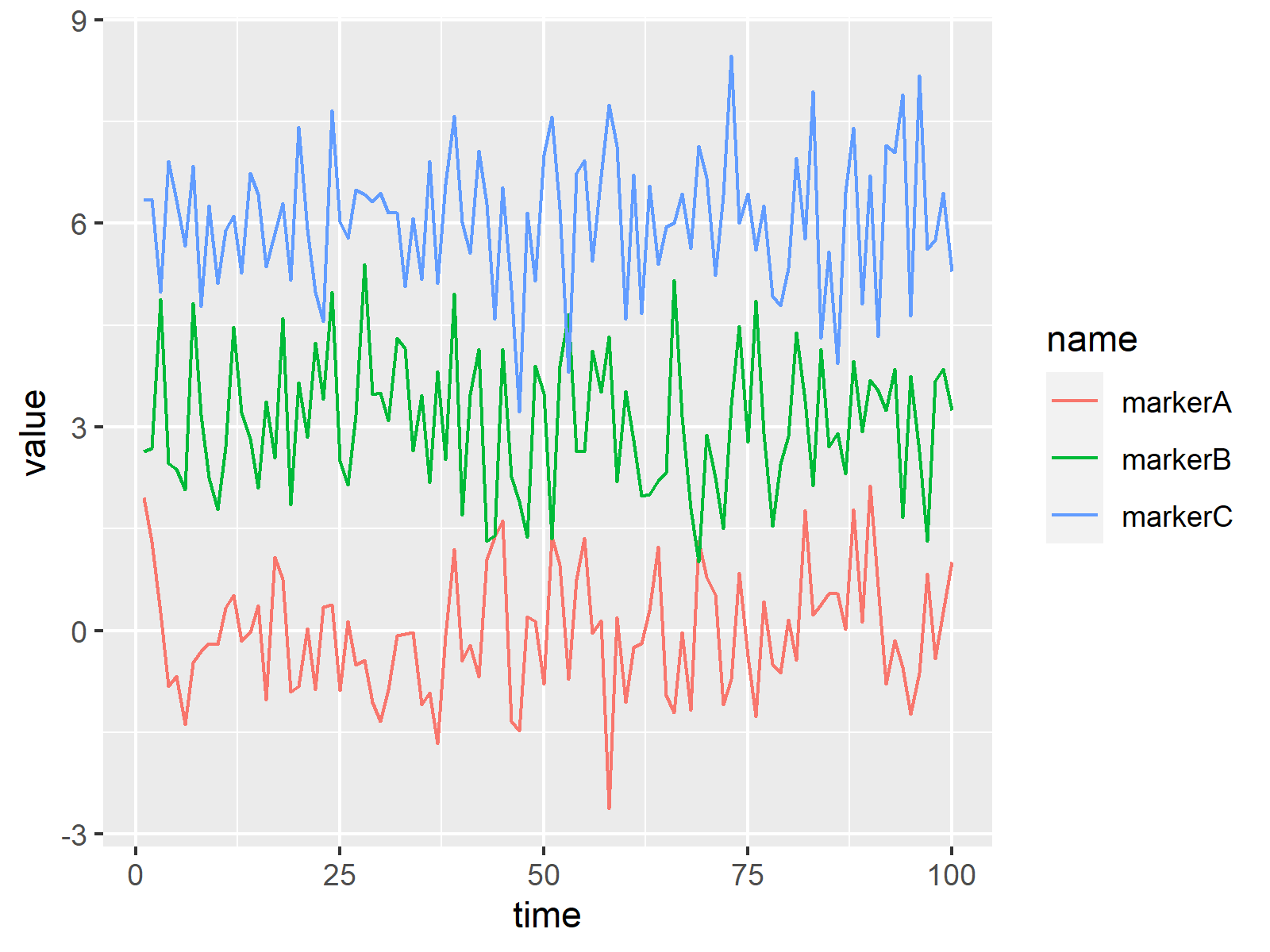You may read more on how to draw multiple time series lines in the same graph in this tutorial.

I hope this helps!

Joachim

•Niels van Steijn
November 17, 2022 7:02 pm

Hi Joachim,

Unfortunatelly, this is not what I intended..
Maybe an easier way to explain my question.

I want to create the same plot you’ve made. But then with multiple subjects in one graph in stead of markers.
Now I’m looking for an easy way to create the same plot, but then for marker A, B, C etc.

It would save me a lot of time if I don’t have to copy paste a few lines of code and edit the Marker value.

Hope you wan’t to help me once more!

•November 18, 2022 8:09 am

Hi Niels,

Thanks for the further explanations. I’m still uncertain if I got your question correctly. However, is the following code doing what you are looking for? It creates three separate images for the three different markers.

```set.seed(8374636)
data <- data.frame(ID = as.factor(1:5),
time = 1:100,
markerA = rnorm(100, 0),
markerB = rnorm(100, 3),
markerC = rnorm(100, 6))

library("tidyr")

marker_all <- c("markerA", "markerB", "markerC")

data_long <- pivot_longer(data, cols = marker_all)

library("ggplot2")

for(i in 1:length(marker_all)) {

ggp_i <- ggplot(data_long[data_long\$name == marker_all[i], ],
aes(x = time,
y = value,
col = ID)) +
geom_line() +
ggtitle(marker_all[i])
print(ggp_i)
Sys.sleep(2)
}```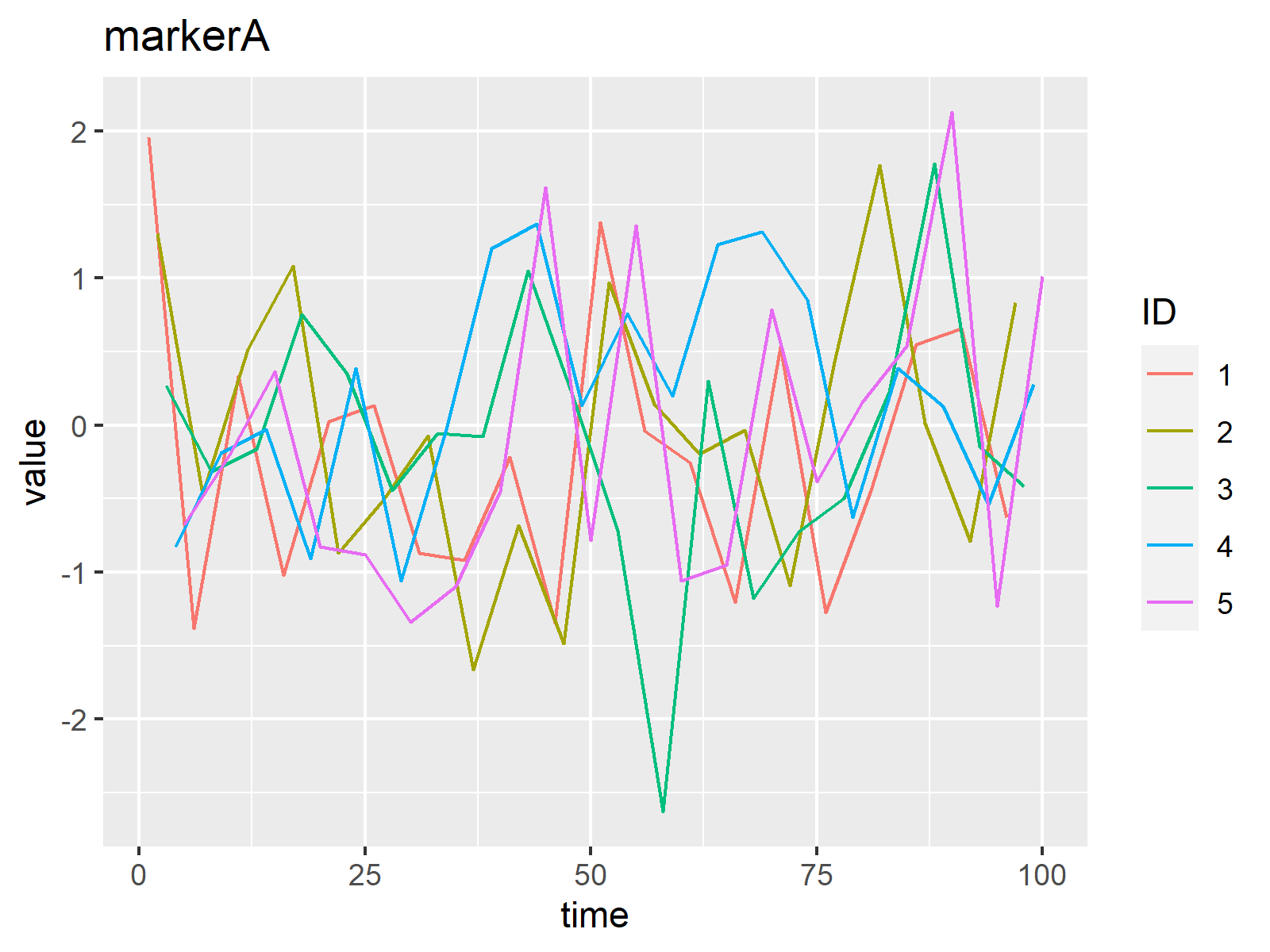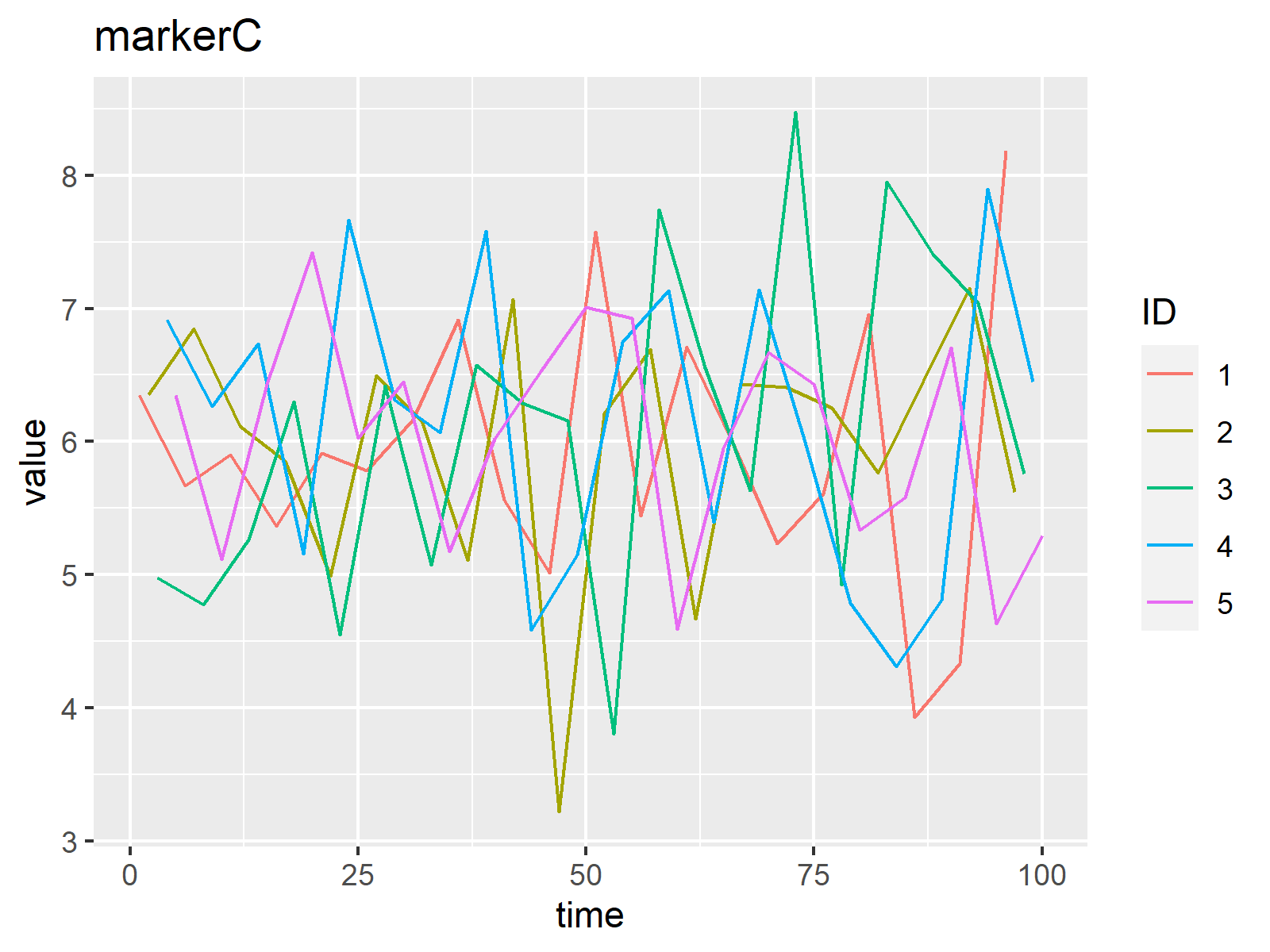Regards,
Joachim

•Niels
November 22, 2022 11:16 am

Hi Joachim,

Yes! I was able to adjust some things, but pivoting to a long format apparently did the trick !
Thanks again!

•November 23, 2022 8:45 am

Hi Niels,

This is great to hear, glad you found a solution!

Regards,
Joachim

•Niels
March 7, 2023 9:35 am

Hi Joachim,

once again I would like to continue on my previous question.
I’m using the loop a lot now, and it works perfectly.

However, now I’ve thought of something new I would like to incorporate, which I can’t figure out how.

Using the same data sample and example as before. Is it possible to highlight some ID’s based on a new value, let’s say Marker D.
For example: Make a dashed line for every ID with Marker D > 10 at timepoint 2, in the plots from Marker A, B C etc.. So the plots from A, B C stay the same, only the linetype would change for some ID’s
Actually, I would be happy with any kind of marking. Different colour, point, line type label etc.

In this way I can easily spot if a participant with a high concentration of Marker D, also has a high concentration of Marker A, B, C etc…

•March 8, 2023 8:21 am

Hello Niels,

I am not sure if I got your question correctly. Do you want to change the line type for all ids for the values which is above the given threshold? Like, change the line type if the value is greater than 10 for all ids and in all plots (Marker A, B, etc.)? Or do you want to do it for only one Marker type? Or do you want to change all graphs Marker A, B, C, etc. yet based on only the values of one Marker, for instance, Marker D? If it is the last one, it might be challenging to implement.

Regards,
Cansu

•Diarmuid MacAmhlaoibh
March 2, 2023 10:11 am

Thank you for this, though I’m not quite making the leap to what I need!
I am hoping to use this, for example, to plot multiple t-distribution curves on the same plot [not a series of plots], where the degrees of freedom are in a vector, and colours are in another vector – alongside a (single) normal distribution plot. I would also like to avoid including the static parts of the plot inside the loop, if possible!

Great set of tutorials and videos — very clear and helpful.
====================================================
library(ggplot2)
dfs = c( 2, 5, 10, 20) # degrees of freedom for ‘t’
cols = rep(c(“red”, “blue”), 2) # colours for plot
n = length(dfs)

for (i in 1:n) {
ggpi <- ggplot(data.frame(x = c(-4, 4)), aes(x)) +
labs(x = "x", y = "", title = "Normal & t distributions") +
scale_x_continuous(breaks=seq(-4,4,1)) +
theme(panel.grid.major=element_line(colour="gray")) +
theme(axis.line.x.bottom=element_line(color="black")) +
theme(axis.line.y.left=element_line(color="black")) +
# normal distribution
stat_function(fun = dnorm, geom = "line", colour="black") +
# series of t dists (this is not working!)
stat_function(fun = dt, args = list(df = dfs[i]),
geom = "line", colour=cols[i])
}
# How to add the ggpi's together?
print(ggpi) # only prints the last loop
===========================================

•Diarmuid MacAmhlaoibh
March 2, 2023 5:33 pm

Got it!!

library(ggplot2)
dfs = c( 2, 5, 10, 20) # degrees of freedom for ‘t’
cols = rep(c(“red”, “blue”), 2) # colours for plot
n = length(dfs)

gg <- ggplot(data.frame(x = c(-4, 4)), aes(x)) +
labs(x = "x", y = "", title = "Normal & t distributions") +
scale_x_continuous(breaks=seq(-4,4,1)) +
theme(panel.grid.major=element_line(colour="gray")) +
theme(axis.line.x.bottom=element_line(color="black")) +
theme(axis.line.y.left=element_line(color="black")) +
# normal distribution
stat_function(fun = dnorm,
args = list(mean = 0, sd = 1),
colour="black",
size = 1)
# t-distribution
for (i in 1:n){
gg <- gg + stat_function(fun = dt,
args = list(df = dfs[i]),
colour = cols[i],
size = 0.8) }
gg

•March 7, 2023 10:30 am

Thank you for your kind words. I am also glad that you fixed it! and thank you for sharing the solution.

Regards,
Cansu

•Diarmuid MacAmhlaoibh
March 2, 2023 8:03 pm

Sorry to be a nuisance!
How would I add a legend, where the relevant degrees of freedom and the colour are picked up from the vector?

•March 7, 2023 10:52 am

Hello Diarmuid,

The legend for colors should be implemented by scale_color_manual normally. However, I also couldn’t achieve that. What about posting your question on our Facebook discussion group? Someone may help you there.

Regards,
Cansu

•Diarmuid MacAmhlaoibh
March 9, 2023 5:52 pm

Many thanks for all your help.
I got it all to work, but had to abandon `stat_function’ and make a dataframe with all the values instead, then using scale_color_manual, scale_linetype_manual and scale_size_manual to get a reasonable-looking legend.
Regards
Diarmuid

•March 14, 2023 4:32 pm

Perfect! Thanks for the update.

Regards,
Cansu

•Niels
March 17, 2023 9:41 pm

Hi Cansu,

But yes, that last suggestion is what I’m looking for.

Take the last three plots that Joachim made in his previous reply.
E.g. I know that ID’s 2, 3 and 5 have a extremely high concentration of Marker D. In order to find relations of Marker D with other Markers, I would like to highlight ID’s 2, 3, and 5 in the plots for Marker A, B and C.

so x = time, y = concentration of marker [i]
group by = ID, colour by = ID, linetype = Marker D > 10

Hope you can help !

•March 18, 2023 10:56 am

Hello Niels,

As an example, I checked the averages by id for marker A and then used another line type for the ID with the highest average, ID=4, in all graphs. See below:

```data_long <- pivot_longer(data, cols = marker_all)
summary<-data_long[data_long\$name=="markerA",]%>%group_by(ID)%>%
summarise_at(vars(value), list(name=mean))
summary

library("ggplot2")

for(i in 1:length(marker_all)) {

ggp_i <- ggplot(data=subset(data_long[data_long\$name == marker_all[i], ], ID!=4),
aes(x = time,
y = value,
col = ID)) + geom_line()+
geom_line(data=subset(data_long[data_long\$name == marker_all[i], ], ID=="4"), linetype="dashed") +
ggtitle(marker_all[i]) + theme_bw()
print(ggp_i)
Sys.sleep(2)
}```

Output for Marker C: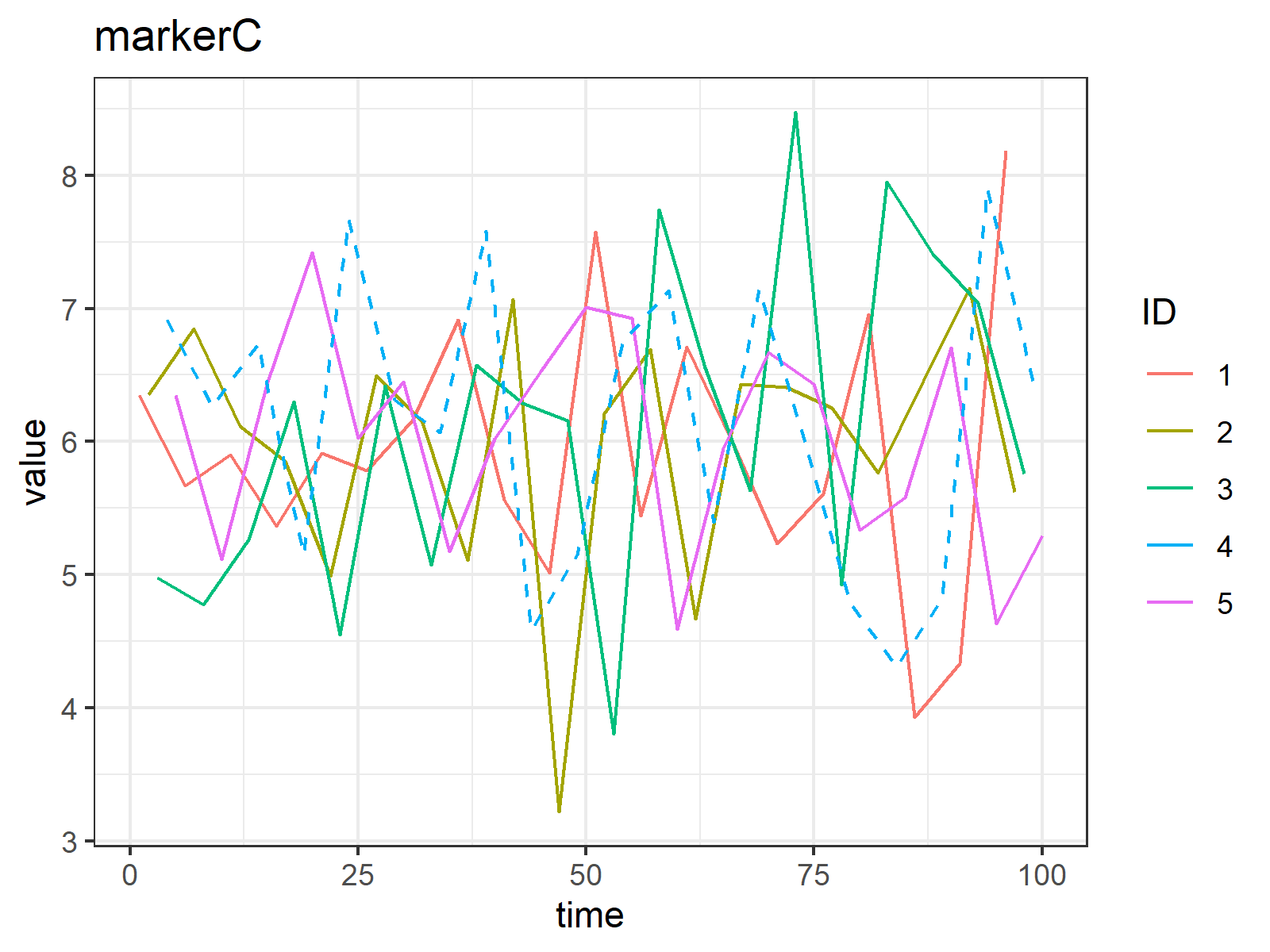The same visual is also created for markers A and B, but I didn’t paste them here; you’ll see them when you run the code. If you are more interested in a more dynamic comparison, separating graphs by ids and labeling the markers would be the easy and reasonable solution. So it will be like this: the first graph is for ID1s only, and each line represents one marker. I think you can adapt the code of Joachim by switching the markers and ids to plot it. Let me know if you want to know something else.

I hope I could help.
Regards,
Cansu

•Niels
March 21, 2023 9:07 am

Hi Cansu,

I think I’m quit happy with this solution, if I would know how to make multiple ID’s with a dashed line. Can you show me, for example, ID’s 4 and 5 as dashed lines ?

•March 21, 2023 10:15 am

Hello Niels,

You can try this:

```for(i in 1:length(marker_all)) {

ggp_i <- ggplot(data=subset(data_long[data_long\$name == marker_all[i], ], ID=="1" | ID=="2" | ID=="3"),
aes(x = time,
y = value,
col = ID)) + geom_line()+
geom_line(data=subset(data_long[data_long\$name == marker_all[i], ], ID=="4" | ID=="5"), linetype="dashed") +
ggtitle(marker_all[i]) + theme_bw()
print(ggp_i)
Sys.sleep(2)
}```

Regards,
Cansu

•Ailin
June 8, 2023 10:17 pm

Hi,
Thank you for the print trick! There is one minor issue though – As I am using GridExtra to combine multiple plots, adding the print command would produce ## TableGrob (1 x 1) “arrange”: 1 grobs
Any ideas about how to get around the printing of TableGrob?

Best,
Ailin

•June 9, 2023 8:40 am

Hello Ailin,

Best,
Cansu

•Raghu
July 15, 2023 5:02 am

> dpd_list
 “DPD 30-3” “DPD 30-6” “DPD 60-6” “DPD 60-9”
my DPD list is above

code:

for(i in 1:length(dpd_list))
{

cat(“\n”)
cat(paste0(“#### “, dpd_list[i], ” {.tabset .tabset-fade} \n”))
cat(“\n”)
report_data_ep2 <- report_data@early_performance_result\$monthly[[dpd_list[i]]]
cat("\n")
cat("\n")
cat(paste0("###### Volume of observed Bad Applications"))
cat("\n")
cat("\n")
(("######"))
cat("\n")

cat("\n")
cat("\n")
}

htmltools::tagList(b)

its giving me last DPD data only in HTML…how to get the data in HTML for all DPD..

•July 17, 2023 8:19 am

Hello,

The issue you’re encountering stems from your loop structure. Within the loop, you are generating some HTML content for each element of your dpd_list. However, only the result from the last iteration of the loop is preserved and passed to htmltools::tagList(). In other words, with each new iteration, you’re overwriting the previous value of b with a new one, thus only keeping the result from the final loop iteration.

To resolve this, you should create a list before the loop begins and then append each plot_pivoted_table() output to this list. Here is the updated code, hopefully it will fix it:

```# Create an empty list to store the results
b <- list()

for(i in 1:length(dpd_list))
{
cat("\n")
cat(paste0("#### ", dpd_list[i], " {.tabset .tabset-fade} \n"))
cat("\n")
report_data_ep2 <- report_data@early_performance_result\$monthly[[dpd_list[i]]]
cat("\n")
cat("\n")
cat(paste0("###### Volume of observed Bad Applications"))
cat("\n")
cat("\n")
cat("######")
# Append each plot to the list